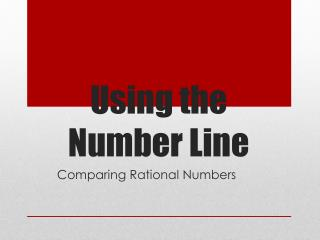DownloadDownload PresentationUsing the Number Line

Using the Number Line

Télécharger la présentationUsing the Number Line

- - - - - - - - - - - - - - - - - - - - - - - - - - - E N D - - - - - - - - - - - - - - - - - - - - - - - - - - -
Presentation Transcript

1. Using the Number Line Comparing Rational Numbers

2. Numbers right of 0 are positive. Numbers left of 0 are negative. Creating a Number Line

3. A number line visually shows how numbers are organized from least to greatest. Numbers get largertoward the right. Reading the Number Line Numbers get smaller toward the left.

4. Numbers get larger going up. A number line visually shows how numbers are organized from least to greatest. Numbers get smaller going down. Reading the Number Line

5. Numbers describe their distance from zero. 3 is three spaces from 0 and -3 is three spaces from 0. 3 and -3 are opposites. Opposites

6. Every number has an opposite. For every positive number, there is a negative number. *Zero is its own opposite! Opposites

7. When comparing numbers use the number line. The number on the right is the bigger number. The number on the left is the smaller number. Ex: -3 is less than -1 -3 < -1 (-3 is on the left of -1 on the number line.) Using the Number Line -3 -1

8. Some students think the greatest number is the number closest to zero. NOPE!  Because that rule is not always true. -5 -2 0 10 -2 is closer to 0 than -5 and is greater than -5… but -2 is closer to 0 than 10 but is less than 10. Common Misconception with Comparing Numbers

9. What is the opposite of the following integers? • 2,345 -2,345 • -16 16 • 0 0 • 8 -8 Using the number line, determine which integer is greater: • 2 -2 > • -5 -7 > • 0 3 < • 0 -8 > Practice

10. means turn Leftor turn Downon the number line. means turn Right or Upon the number line. The signs of a number tell which way to go on the number line, like a GPS gives directions! Signs of Numbers - GPS